# Explain The Logic NAND Gate With its Operation and How it Works as A Universal Gate

## NAND Gate

The term NAND is a contraction of NOT-AND and implies an AND function with complemented (inverted) output.  In this gate, output is low only when all inputs are high. A standard logic symbol for a 2-input NAND gate and the equivalent AND gate followed by an INVERTER are shown in fig 1.

Fig 1 (a)                                                                             Fig 1(b)

The standard symbol for the NAND gate is the same as the AND gate symbol except for small circle on its output. This small circle denotes the inverse operation. Thus the NAND gate operated like an AND gate followed by an INVERTER ,so that the circuits shown in fig 1(a) and (b) are equivalent.

The operation of this gate can be described as below :

The output of the AND gate Z’ can be written as :

Z’ = A.B.C…….N

And the output of the NOT gate can be written as :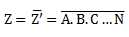The logical operation represented by above equation  is known as NAND operation.

### Truth Table of NAND Gate

The truth table for 2-input NAND gate is given in table 1.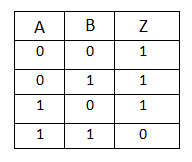Table 1

From table 1 we find that NAND gate output is the exact inverse of the AND gate for all possible input conditions. The NAND gate output goes low only when all the inputs are high while the AND gate output goes high only when all the inputs are high.

The logic equation for NAND gate is given as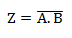and is read as’ Z equals NOT (A AND B)’.

### Diode-Transistor Circuit of NAND Gate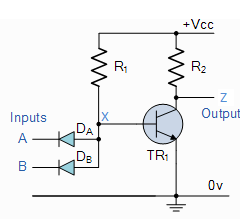Fig 2

Here a diode AND circuit is connected to a transistor NOT circuit that gives the NAND circuit.

It can be seen from the fig 2 that the point X would be driven to ground when either of the  diodes DA or DB or both DA and DB conduct. Under such conditions, the transistor is cutoff and, therefore, output goes high. Output is low only when both input voltages are high (at +VCC) so that X is at +VCC and the transistor operated in saturation.

### NAND gate using OR Gate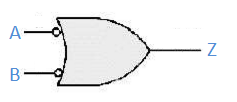Fig 3

Fig 3 shows an OR gate with its inputs inverted .

It acts as a NAND gate.

If we look at table 2 (truth table for OR gate with its inputs inverted), we find that it is the same truth table for NAND gate (table 1).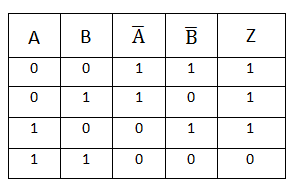Table 2

So an OR gate with its inputs inverted can be used as a NAND gate.

### NAND as Universal Gate

In general, the Logic NAND Gate is classed as a “Universal” gate since it is one of the most commonly used logic gate types.

NAND gates can also be used to produce any other type of logic gate function, and in practice the NAND gate forms the basis of most practical logic circuits.

By connecting the NAND gates together in various combinations the three basic gate types of AND, OR and NOT function can be formed .

### Various Logic Gates using only NAND Gates

The three logic operations NOT, OR and AND can be performed by using only the NAND gates, as shown below .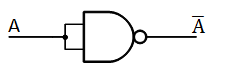Fig 4

NOT logic operation can be performed by tying its two inputs together, as shown in fig 4 .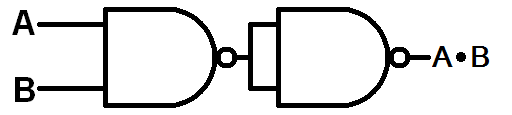Fig 5

AND logic operation can be performed by using two NAND gates, as shown in fig 5 .Fig 6

OR logic operation can be performed by three NAND gates, as shown in fig 6 .

### Standard Package

Fig 7 depicts the pin diagram of an IC 7400 a TTL, quad 2-input NAND gate.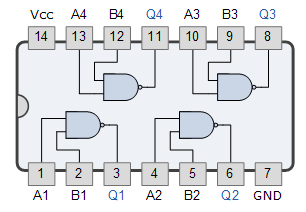Fig 7

It contains four 2-input NAND gates inside a 14-pin DIP.

### Pulsed Operation

The pulsed operation of the NAND gate is shown in fig 8 .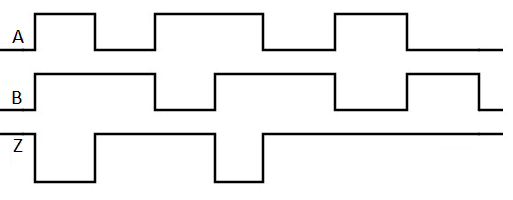Fig 8

The output can be determined in several ways. One way is to draw the output for AND gate, and then invert it. Another way is by using truth table of NAND gate.

As we can see from the above pulsed waveforms , when both the inputs A and B are high the output Z is low and when either or both inputs are low, the output is high.